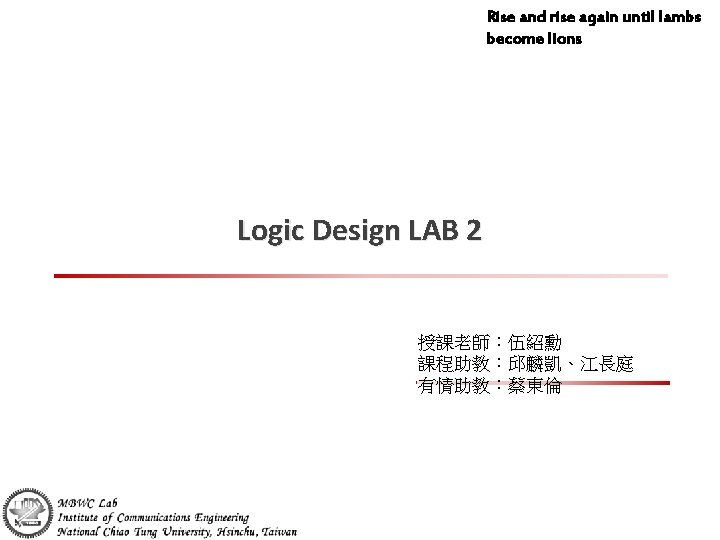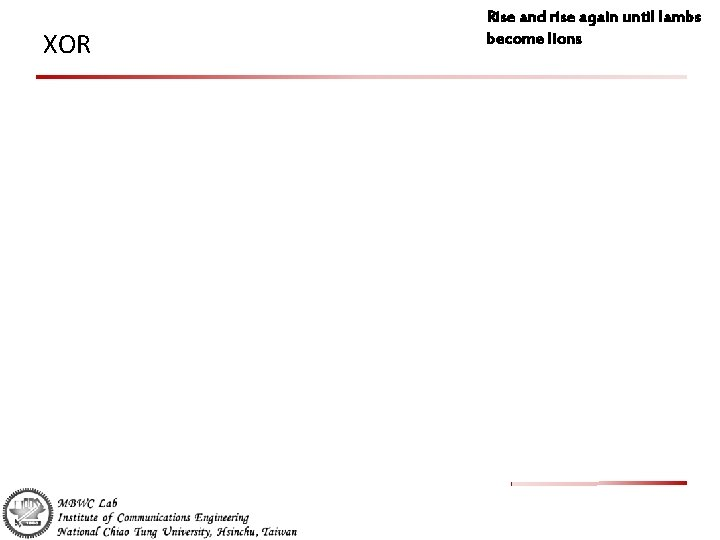# Rise and rise again until lambs become lions

• Slides: 8Rise and rise again until lambs become lions Logic Design LAB 2 授課老師：伍紹勳 課程助教：邱麟凱、江長庭 有情助教：蔡東倫Outlines Rise and rise again until lambs become lions Familiar how to put theory into practice NAND – Boolean algebra representation of NAND – Truth table of NAND XOR – Boolean algebra representation of XOR – Truth table of XOR LAB 2 – Implement XOR by NAND gateExperiment materials Bread board Power supply IC: 7400*2 Light-emitting diode (LED)*2 Rise and rise again until lambs become lionsNAND Gate (7400 IC) Rise and rise again until lambs become lions Boolean algebra representation of NAND – Y= (AB)’ Truth table of NAND Input A Input B Output Y 0 0 1 1 1 0Rise and rise again until lambs become lions XOR Boolean algebra representation of XOR – Y = A⊕B = AB’+A’B (sum of product) Truth table of XOR Input A Input B Output Y 0 0 1 1 1 0XOR Rise and rise again until lambs become lionsLAB 2 Rise and rise again until lambs become lions Implement XOR by NAND gate – Y = A⊕B = AB’+A’B Most of all digital circuits can be implemented by NAND gates – – NOT gate: A’ = (AA)’ AND gate: AB = ( (AB)’ )’ OR gate: A+B = ( (A’)(B’) )’ (De Morgan's law) Combinational logic: AB+CD = ( (AB)’(CD)’ )’LAB 2 Implement XOR by NAND gate – Y = A⊕B = AB’+A’B Rise and rise again until lambs become lions